International
Tables for
Crystallography
Volume B
Reciprocal space
Edited by U. Shmueli

International Tables for Crystallography (2006). Vol. B, ch. 5.1, pp. 536-537   | 1 | 2 |

## Section 5.1.2.5. Dispersion surface

A. Authiera*

aLaboratoire de Minéralogie-Cristallographie, Université P. et M. Curie, 4 Place Jussieu, F-75252 Paris CEDEX 05, France
Correspondence e-mail: authier@lmcp.jussieu.fr

#### 5.1.2.5. Dispersion surface

| top | pdf |

The fundamental equations (5.1.2.20)of dynamical theory are a set of linear homogeneous equations whose unknowns are the amplitudes of the various waves which make up a wavefield. For the solution to be non-trivial, the determinant of the set must be set equal to zero. This provides a secular equation relating the magnitudes of the wavevectors of a given wavefield. This equation is that of the locus of the tie points of all the wavefields that may propagate in the crystal with a given frequency. This locus is called the dispersion surface. It is a constant-energy surface and is the equivalent of the index surface in optics. It is the X-ray analogue of the constant-energy surfaces known as Fermi surfaces in the electron band theory of solids.

In the two-beam case, the dispersion surface is a surface of revolution around the diffraction vector OH. It is made from two spheres and a connecting surface between them. The two spheres are centred at O and H and have the same radius, nk. Fig. 5.1.2.3shows the intersection of the dispersion surface with a plane passing through OH. When the tie point lies on one of the two spheres, far from their intersection, only one wavefield propagates inside the crystal. When it lies on the connecting surface, two waves are excited simultaneously. The equation of this surface is obtained by equating to zero the determinant of system (5.1.2.20):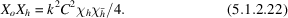Figure 5.1.2.3 | top | pdf |Intersection of the dispersion surface with the plane of incidence. The dispersion surface is a connecting surface between the two spheres centred at reciprocal-lattice points O and H and with radius nk.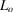is the Lorentz point.

Equations (5.1.2.21)show that, in the zero-absorption case,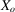and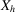are to be interpreted as the distances of the tie point P from the spheres centred at O and H, respectively. From (5.1.2.20)it can be seen that they are of the order of the vacuum wavenumber times the Fourier coefficient of the dielectric susceptibility, that is five or six orders of magnitude smaller than k. The two spheres can therefore be replaced by their tangential planes. Equation (5.1.2.22)shows that the product of the distances of the tie point from these planes is constant. The intersection of the dispersion surface with the plane passing through OH is therefore a hyperbola (Fig. 5.1.2.4) whose diameter [using (5.1.2.5)and (5.1.2.22)] is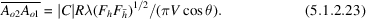Figure 5.1.2.4 | top | pdf |Intersection of the dispersion surface with the plane of incidence shown in greater detail. The Lorentz pointis far away from the nodes O and H of the reciprocal lattice: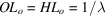is about 105 to 106 times larger than the diameter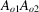of the dispersion surface.

It can be noted that the larger the diameter of the dispersion surface, the larger the structure factor, that is, the stronger the interaction of the waves with the matter. When the polarization is parallel to the plane of incidence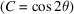, the interaction is weaker.

The asymptotes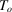andto the hyperbola are tangents to the circles centred at O and H, respectively. Their intersection,, is called the Lorentz point (Fig. 5.1.2.4).

A wavefield propagating in the crystal is characterized by a tie point P on the dispersion surface and two waves with wavevectors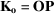and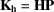, respectively. The ratio, ξ, of their amplitudes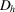and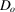is given by means of (5.1.2.20):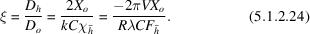The hyperbola has two branches, 1 and 2, for each direction of polarization, that is, for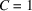or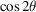(Fig. 5.1.2.5). Branch 2 is the one situated on the same side of the asymptotes as the reciprocal-lattice points O and H. Given the orientation of the wavevectors, which has been chosen away from the reciprocal-lattice points (Fig. 5.1.2.1b), the coordinates of the tie point,and, are positive for branch 1 and negative for branch 2. The phase of ξ is therefore equal to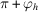and tofor the two branches, respectively, whereis the phase of the structure factor [equation (5.1.2.6)]. This difference of π between the two branches has important consequences for the properties of the wavefields.Figure 5.1.2.5 | top | pdf |Dispersion surface for the two states of polarization. Solid curve: polarization normal to the plane of incidence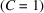; broken curve: polarization parallel to the plane of incidence. The direction of propagation of the energy of the wavefields is along the Poynting vector, S, normal to the dispersion surface.

As mentioned above, owing to absorption, wavevectors are actually complex and so is the dispersion surface.